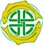﻿ Similarity and Distance MeasuresSimilarity and Distance Measures

Choose the similarity measure you wish to calculate from the Similarity drop-down menu. The calculations will appear on the Similarity tab at the bottom of the program window. For ease of use, the program will highlight sites with similarity above a certain level. You can set this level by entering the number in the Set threshold level box at the bottom of the page.

The similarity measures used.

These are simple measures of either the extent to which two habitats have species in common (Q analysis) or which variables (species) have habitats in common (R analysis ). Binary similarity coefficients use presence-absence data; following the introduction of computers, more complex quantitative coefficients became practicable. Analysis of quantitative, rather than presence-absence, data with a binary method may report a perfect similarity between every sample/site in data sets (such as the Romano British pottery demo data set) in which each variable is present in every sample.

Both groups of indices can be further divided between those which take account of the absence from both communities (double zero methods) and those which do not. In most ecological applications it is unwise to use double-zero methods as they assign a high level of similarity to localities which both lack many species; a problem which becomes particularly acute in habitats which have a potentially extremely large species list, such as the marine benthos.

A good account of similarity and distance measures is given in Legendre & Legendre (1983). Because of division by zero problems for some data sets not all measures can be calculated. When a division by zero error would occur CAP gives an index of -99.

For measures of similarity between samples based on species presence-absence, the observations can be summarised in a simple frequency table:

 Sample 1 Species Present Species Absent Sample 2 Species Present a b Species  Absent c d

where the number of species present in both samples is a, the number of species present in sample 1 but missing from sample 2 is b, the number of species missing in sample 1 but present in sample 2 is c and the number of species missing from both samples is d. The total number of species, N, is therefore a+b+c+d.

Binary - double zeros

Simple matching

Rogers_Tanimoto

S3

S4

S5

S6

Binary - no double zeros

Jaccards

Sørensen

S9

S10

Russel & Rao

Kulczynski

S13

Ochiai

Quantitative

Q1

Q2

Steinhaus

Kulczynski-Quantitative

Distance measures

Euclidean

Mahalanobis

Average

Chord

Geodesic

Manhattan

Mean character difference

Whittaker

Canberra

Bray-Curtis

Renkonen